#### Analyses in the MULTREG Statement

##### Type III F Test in Multiple Regression (TEST=TYPE3)

Maxwell (2000) discusses a number of different ways to represent effect sizes (and to compute exact power based on them) in multiple regression. PROC POWER supports two of these, multiple partial correlation and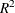in full and reduced models.

Let p denote the total number of predictors in the full model (excluding the intercept), and let Y denote the response variable. You are testing that the coefficients of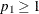predictors in a set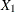are 0, controlling for all of the other predictors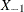, which consists of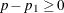variables.

The hypotheses can be expressed in two different ways. The first is in terms of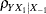, the multiple partial correlation between the predictors inand the response Y adjusting for the predictors in: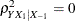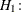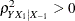The second is in terms of the multiple correlations in full (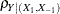) and reduced (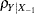) nested models: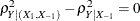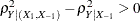Note that the squared values ofandare the populationvalues for full and reduced models.

The test statistic can be written in terms of the sample multiple partial correlation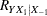,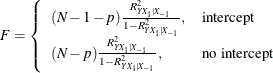or the sample multiple correlations in full (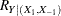) and reduced () models,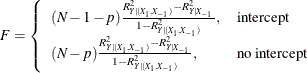The test is the usual Type III F test in multiple regression: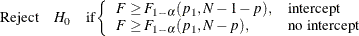Although the test is invariant to whether the predictors are assumed to be random or fixed, the power is affected by this assumption. If the response and predictors are assumed to have a joint multivariate normal distribution, then the exact power is given by the following formula: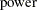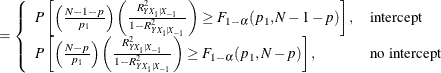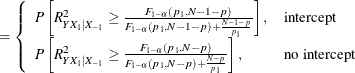The distribution of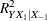(for any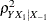) is given in Chapter 32 of Johnson, Kotz, and Balakrishnan (1995). Sample size tables are presented in Gatsonis and Sampson (1989).

If the predictors are assumed to have fixed values, then the exact power is given by the noncentral F distribution. The noncentrality parameter is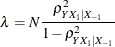or equivalently,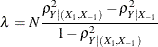The power is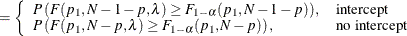The minimum acceptable input value of N depends on several factors, as shown in Table 71.30.

Table 71.30: Minimum Acceptable Sample Size Values in the MULTREG Statement

Predictor Type

Intercept in Model?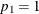?

Minimum N

Random

Yes

Yes

p + 3

Random

Yes

No

p + 2

Random

No

Yes

p + 2

Random

No

No

p + 1

Fixed

Yes

Yes or No

p + 2

Fixed

No

Yes or No

p + 1